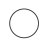# [hard core dry goods] data type conversion in pandas module

2022-06-24 08:14:03

When we are sorting out the data , Data type errors often occur , Today, I'd like to share with you about `Pandas` Module in the data type conversion related skills , It's full of dry goods ！

## Import datasets and modules

So our first routine is to import Pandas Module and create data set , The code is as follows

``````import pandas as pd
import numpy as np

df = pd.DataFrame({
'string_col': ['1','2','3','4'],
'int_col': [1,2,3,4],
'float_col': [1.1,1.2,1.3,4.7],
'mix_col': ['a', 2, 3, 4],
'missing_col': [1.0, 2, 3, np.nan],
'money_col': ['£1,000.00', '£2,400.00', '£2,400.00', '£2,400.00'],
'boolean_col': [True, False, True, True],
'custom': ['Y', 'Y', 'N', 'N']
})

df``````

outputLet's first look at the data types of each column , The code is as follows

``df.dtypes``

output

``````string_col      object
int_col          int64
float_col      float64
mix_col         object
missing_col    float64
money_col       object
boolean_col       bool
custom          object
dtype: object``````

Of course, we can also call info() Method to achieve the above purpose , The code is as follows

``df.info()``

output

``````<class 'pandas.core.frame.DataFrame'>
RangeIndex: 4 entries, 0 to 3
Data columns (total 8 columns):
#   Column       Non-Null Count  Dtype
---  ------       --------------  -----
0   string_col   4 non-null      object
1   int_col      4 non-null      int64
2   float_col    4 non-null      float64
3   mix_col      4 non-null      object
4   missing_col  3 non-null      float64
5   money_col    4 non-null      object
6   boolean_col  4 non-null      bool
7   custom       4 non-null      object
dtypes: bool(1), float64(2), int64(1), object(4)
memory usage: 356.0+ bytes``````

## Data type conversion

Next, we start the data type conversion , The most commonly used is `astype()` Method , For example, we convert floating-point data to integer , The code is as follows

``df['float_col'] = df['float_col'].astype('int')``

Or we'll take one of them “string_col” This column is converted to integer data , The code is as follows

``df['string_col'] = df['string_col'].astype('int')``

Of course, we consider from the perspective of saving memory , convert to `int32` perhaps `int16` Data of type ,

``````df['string_col'] = df['string_col'].astype('int8')
df['string_col'] = df['string_col'].astype('int16')
df['string_col'] = df['string_col'].astype('int32')``````

Then let's take a look at the data types of each column after conversion

``df.dtypes``

output

``````string_col     float32
int_col          int64
float_col        int32
mix_col         object
missing_col    float64
money_col       object
boolean_col       bool
custom          object
dtype: object``````

But when a column has more than one data type , An error will be reported during the conversion process , for example “mix_col” This column

``df['mix_col'] = df['mix_col'].astype('int')``

output

``ValueError: invalid literal for int() with base 10: 'a'``

So we can call `to_numeric()` Methods and `errors` Parameters , The code is as follows

``````df['mix_col'] = pd.to_numeric(df['mix_col'], errors='coerce')
df``````

outputAnd if you encounter missing values , An error will also occur during data type conversion , The code is as follows

``df['missing_col'].astype('int')``

output

``ValueError: Cannot convert non-finite values (NA or inf) to integer``

We can start by calling fillna() Method to populate missing values with other values , And then type conversion , The code is as follows

``````df["missing_col"] = df["missing_col"].fillna(0).astype('int')
df``````

outputAnd finally “money_col” This column , We can see the currency symbol in it , So the first step we have to do is to replace these currency symbols , Then the data type is converted , The code is as follows

``````df['money_replace'] = df['money_col'].str.replace('£', '').str.replace(',','')
df['money_replace'] = pd.to_numeric(df['money_replace'])
df['money_replace']``````

output

``````0    1000.0
1    2400.0
2    2400.0
3    2400.0``````

## When encountering time series data

When we need to type convert data in date format , What you usually need to call is `to_datetime()` Method , The code is as follows

``````df = pd.DataFrame({'date': ['3/10/2015', '3/11/2015', '3/12/2015'],
'value': [2, 3, 4]})
df``````

outputLet's first look at the data types of each column

``df.dtypes``

output

``````date     object
value     int64
dtype: object``````

We call `to_datetime()` The code of the method is as follows

``pd.to_datetime(df['date'])``

output

``````0   2015-03-10
1   2015-03-11
2   2015-03-12
Name: date, dtype: datetime64[ns]``````

Of course, this does not mean that you cannot call `astype()` The method , The result is the same as the above , The code is as follows

``df['date'].astype('datetime64')``

When we encounter date format data in user-defined format , Also call `to_datetime()` Method , But the format that needs to be set is `format` Parameters need to be consistent

``````df = pd.DataFrame({'date': ['2016-6-10 20:30:0',
'2016-7-1 19:45:30',
'2013-10-12 4:5:1'],
'value': [2, 3, 4]})

df['date'] = pd.to_datetime(df['date'], format="%Y-%d-%m %H:%M:%S")``````

output## Is it possible to achieve the goal in one step ？

Last , Maybe someone will ask , Is there any way to realize data type conversion in one step ？ That, of course, can be achieved , The code is as follows

``````df = pd.DataFrame({'date_start': ['3/10/2000', '3/11/2000', '3/12/2000'],
'date_end': ['3/11/2000', '3/12/2000', '3/13/2000'],
'string_col': ['1','2','3'],
'float_col': [1.1,1.2,1.3],
'value': [2, 3, 4]})

df = df.astype({
'date_start': 'datetime64',
'date_end': 'datetime64',
'string_col': 'int32',
'float_col': 'int64',
'value': 'float32',
})``````

Let's take a look at the results

``df``

outputNO.1

Previous recommendation

Historical articles

Share 、 Collection 、 give the thumbs-up 、 I'm looking at the arrangement ？